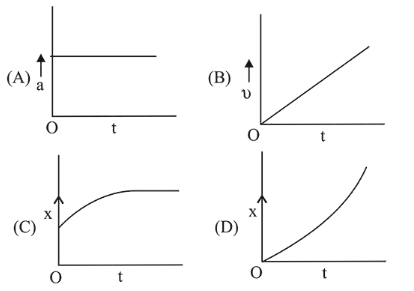# A particle starts from origin O from rest and moves with a uniform acceleration along the positive x-axis.Question:

A particle starts from origin $\mathrm{O}$ from rest and moves with a uniform acceleration along the positive $x$-axis. Identify all figures that correctly represents the motion qualitatively ( $a=$ acceleration, $v=$ velocity,$x=$ displacement $t=$ time $)$1. (1) (B), (C)

2. (2) (A)

3. (3) (A), (B), (C)

4. (4) (A), (B), (D)

Correct Option: , 4

Solution:

(4) For constant acceleration, there is straight line parallel to t-axis on $\vec{a}-t$.

Inclined straight line on $\vec{v}-t$, and parabola on $\vec{x}-t$.